# A student

A student is to answer 8 out of 10 questions on the exam.
a) find the number n of ways the student can choose 8 out of 10 questions
b) find n if the student must answer the first three questions
c) How many if he must answer at least 4 of the first 5 questions?

Result

a =  45
b =  21
c =  35

#### Solution:Leave us a comment of example and its solution (i.e. if it is still somewhat unclear...):

Showing 0 comments:Be the first to comment!#### To solve this verbal math problem are needed these knowledge from mathematics:

Would you like to compute count of combinations?

## Next similar examples:

1. Mumbai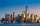A job placement agency in Mumbai had to send ten students to five companies two to each. Two of the companies are in Mumbai and others are outside. Two of the students prefer to work in Mumbai while three prefer to work outside. In how many ways assignment
2. TrinityHow many different triads can be selected from the group 43 students?
3. A bookA book contains 524 pages. If it is known that a person will select any one page between the pages numbered 125 and 384, find the probability of choosing the page numbered 252 or 253.
4. MenuOn the menu are 12 kinds of meal. How many ways can we choose four different meals into the daily menu?
5. Fish tankA fish tank at a pet store has 8 zebra fish. In how many different ways can George choose 2 zebra fish to buy?
6. First class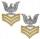The shipment contains 40 items. 36 are first grade, 4 are defective. How many ways can select 5 items, so that it is no more than one defective?
7. Disco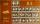On the disco goes 12 boys and 15 girls. In how many ways can we select four dancing couples?
8. TeamsHow many ways can divide 16 players into two teams of 8 member?
9. Weekly serviceIn the class are 20 pupils. How many opportunities have the teacher if he wants choose two pupils randomly who will weeklies?
10. DivisionDivision has 18 members: 10 girls and 6 boys, 2 leaders. How many different patrols can be created, if one patrol is 2 boys, 3 girls and 1 leader?
11. Calculation of CNCalculate: ?
12. Theorem proveWe want to prove the sentence: If the natural number n is divisible by six, then n is divisible by three. From what assumption we started?
13. 2nd class combinationsFrom how many elements you can create 4560 combinations of the second class?
14. Count of trianglesGiven a square ABCD and on each side 8 internal points. Determine the number of triangles with vertices at these points.
15. Hockey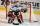Hockey match ended 8:2. How many different matches could be?
16. PIN - codesHow many five-digit PIN - code can we create using the even numbers?
17. Hearts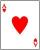5 cards are chosen from a standard deck of 52 playing cards (13 hearts) with replacement. What is the probability of choosing 5 hearts in a row?